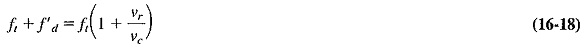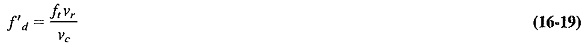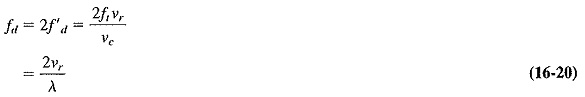The apparent frequency of electromagnetic or sound waves depends on the relative radial motion of the source and the observer. If source and observer are moving away from each other, the apparent frequency will decrease, while if they are moving toward each other, the apparent frequency will increase. This was postulated in 1842 by Christian Doppler and put on a firm mathematical basis by Armand Fizeau in 1848, The Doppler Effect in Radar is observable for light and is responsible for the so-called red shift of the spectral lines from stellar objects moving away from the solar system. It is equally noticeable for sound, being the cause of the change in the pitch of a whistle from a passing train. It can also be used to advantage in several forms of radar.

Consider an observer situated on a platform approaching a fixed source of radiation, with a relative velocity +νr. A stationary observer would note ft wave crests (or troughs) per second if the transmitting frequency were ft. Because the observer is moving toward the source, that person of course encounters more than ft crests per second. The number observed under these conditions is given byConsequently,where

ft + f′d = new observed frequency

f′d = Doppler frequency difference

Note that the foregoing holds if the relative velocity, νr, is less than about 10 percent of the velocity of light, νc. If the relative velocity is higher than that (most unlikely in practical cases), relativistic effects must be taken into account, and a somewhat more complex formula must be applied. The principle still holds under those conditions, and it holds equally well if the observer is stationary and the source is in motion. Equation (16-19) was calculated for a positive radial velocity, but if νr is negative, f′in Equation (16-19) merely acquires a negative sign. In radar involving a moving target, the signal undergoes the Doppler shift when impinging upon the target. This target becomes the “source” of the reflected waves, so that we now have a moving source and a stationary observer (the radar receiver). The two are still approaching each other, and so the Doppler Effect in Radar is encountered a second time, and the overall effect is thus double.

Hence the Doppler frequency for radar issince ftc =1/λ, where λ is the transmitted wavelength.

The same magnitude of Doppler shift is observed regardless of whether a target is moving toward the radar or away from it, with a given velocity. However, it will represent an increase in frequency in the former case and .a reduction in the latter. Note also that the Doppler Effect in Radar is observed only for radial motion, not for tangential motion. Thus no Doppler Effect in Radar will be noticed if a target moves across the field of view of a radar.

However, a Doppler shift will be apparent if the target is rotating, and the resolution of the radar is sufficient to distinguish its leading edge from its trailing edge. One example where this has been employed is the measurement of the rotation of the planet Venus (whose rotation cannot be observed by optical telescope because of the very dense cloud cover).

On the basis of this frequency change, it is possible to determine the relative velocity of the target, with either pulsed or CW radar, as will be shown. One can also distinguish between stationary and moving targets and eliminate the blips due to stationary targets. This may be done with pulsed radar by using moving-target indication.

Scroll to Top